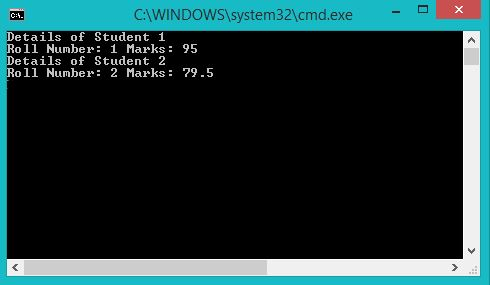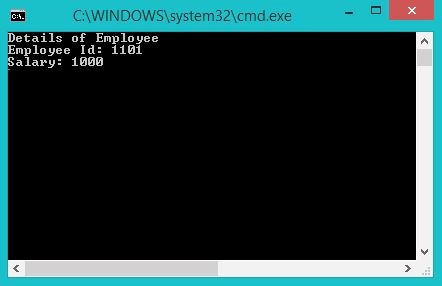# How to access structure elements using Pointers in C#

Unlike C/C++, Structures in C# can have members that are methods, fields, indexers, operator methods, properties or events. The members can have access specifiers as public, private, and internal.
Pointers are variables that store the addresses of the same type of variable i.e. an int pointer can store an address of an integer, a char pointer can store an address of a char and similarly for all other data types, fundamental or user-defined.

You can access a structure member using pointers, of type structure, in the following ways;

1) Using the arrow operator: If the members of the structure are public then you can directly access them using the arrow operator ( -> ). If they are private then you can define methods for accessing the values and use pointers to access the methods. The arrow operator can be used to access structure variables as well as methods.

Syntax:

`PointerName->memberName;`

Example:

 `// C# Program to show the use of  ` `// pointers to access struct members ` `using` `System; ` ` `  `namespace` `GFG { ` ` `  `// Defining a struct Student ` `struct` `Student ` `{ ` `    ``// With members ` `    ``// roll number and marks ` `    ``public` `int` `rno; ` `    ``public` `double` `marks; ` ` `  `    ``// Constructor to initialize values ` `    ``public` `Student(``int` `r, ``double` `m) ` `    ``{ ` `        ``rno = r; ` `        ``marks = m; ` `    ``} ` `}; ``// end of struct Student ` ` `  `class` `Program { ` ` `  `    ``// Main Method ` `    ``static` `void` `Main(``string``[] args) ` `    ``{ ` `        ``// unsafe so as to use pointers ` `        ``unsafe` `        ``{ ` `            ``// Declaring two Student Variables ` `            ``Student S1 = ``new` `Student(1, 95.0); ` `            ``Student S2 = ``new` `Student(2, 79.5); ` ` `  `            ``// Declaring two Student pointers ` `            ``// and initializing them with addresses ` `            ``// of S1 and S2 ` `            ``Student* S1_ptr = &S1; ` `            ``Student* S2_ptr = &S2; ` ` `  `            ``// Displaying details of Student using pointers ` `            ``// Using  the arrow ( -> ) operator ` `            ``Console.WriteLine(``"Details of Student 1"``); ` ` `  `            ``Console.WriteLine(``"Roll Number: {0} Marks: {1}"``,  ` `                            ``S1_ptr -> rno, S1_ptr -> marks); ` ` `  `            ``Console.WriteLine(``"Details of Student 2"``); ` `            ``Console.WriteLine(``"Roll Number: {0} Marks: {1}"``, ` `                            ``S2_ptr -> rno, S2_ptr -> marks); ` ` `  `        ``} ``// end unsafe ` ` `  `    ``} ``// end main ` ` `  `} ``// end class ` ` `  `} `

Output:2) Using Dereferencing operator: You can also access structure elements using the dereferencing operator on the pointer, which is using an asterisk to dereference the pointer and then using the dot operator to specify the structure element.

Syntax:

`(*PointerName).MemberName;`

Example:

 `// C# Program to illustrate the use  ` `// of dereferencing operator ` `using` `System; ` ` `  `namespace` `GFG { ` ` `  `// Defining struct Employee ` `struct` `Employee{ ` ` `  `    ``// Elements Eid and Salary ` `    ``// With properties get and set ` `    ``public` `int` `Eid  ` `    ``{      ` `        ``get``; ` `        ``set``; ` `    ``} ` ` `  `public` `double` `Salary ` `{ ` `    ``get``; ` `    ``set``; ` `} ` `} ` `; ``// Employee ends ` ` `  `class` `Program { ` ` `  `    ``// Main Method ` `    ``static` `void` `Main(``string``[] args) ` `    ``{ ` `        ``// unsafe so as to use pointers ` `        ``unsafe` `        ``{ ` `            ``// Declaring a variable of type Employee ` `            ``Employee E1; ` ` `  `            ``// Declaring pointer of type Employee ` `            ``// initialized to point to E1 ` `            ``Employee* ptr = &E1; ` ` `  `            ``// Accessing struct elements using ` `            ``// dereferencing operator ` `            ``// calls the set accessor ` `            ``(*ptr).Eid = 1101; ` `            ``(*ptr).Salary = 1000.00; ` ` `  `            ``Console.WriteLine(``"Details of Employee"``); ` `            ``// calls the get accessor ` `            ``Console.WriteLine(``"Employee Id: {0}\nSalary: {1}"``, ` `                                   ``(*ptr).Eid, (*ptr).Salary); ` ` `  `        ``} ``// end unsafe ` ` `  `    ``} ``// end Main ` ` `  `} ``// end class ` ` `  `} `

Output:Note: To compile unsafe code on Visual Studio (2012), Go to Project –> ProjectName Properties –> Build –> Check the “Allow unsafe code” box.

My Personal Notes arrow_drop_upStudent at Padre Conceicao College of Engineering, Goa Computer Engineering

If you like GeeksforGeeks and would like to contribute, you can also write an article using contribute.geeksforgeeks.org or mail your article to contribute@geeksforgeeks.org. See your article appearing on the GeeksforGeeks main page and help other Geeks.

Please Improve this article if you find anything incorrect by clicking on the "Improve Article" button below.

Article Tags :

1

Please write to us at contribute@geeksforgeeks.org to report any issue with the above content.